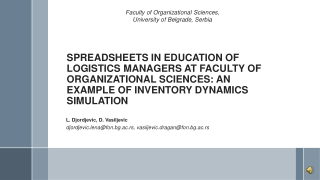DownloadDownload PresentationL. Djordjevic , D. Vasiljevic djordjevic.lena@fon.bg.ac.rs, vasiljevic.dragan@fon.bg.ac.rs

# L. Djordjevic , D. Vasiljevic djordjevic.lena@fon.bg.ac.rs, vasiljevic.dragan@fon.bg.ac.rs

Download Presentation## L. Djordjevic , D. Vasiljevic djordjevic.lena@fon.bg.ac.rs, vasiljevic.dragan@fon.bg.ac.rs

- - - - - - - - - - - - - - - - - - - - - - - - - - - E N D - - - - - - - - - - - - - - - - - - - - - - - - - - -
##### Presentation Transcript

1. Faculty of Organizational Sciences, University of Belgrade, Serbia SPREADSHEETS IN EDUCATION OF LOGISTICS MANAGERS AT FACULTY OF ORGANIZATIONAL SCIENCES: AN EXAMPLE OF INVENTORY DYNAMICS SIMULATION L. Djordjevic, D. Vasiljevic djordjevic.lena@fon.bg.ac.rs, vasiljevic.dragan@fon.bg.ac.rs

2. Agenda • Spreadsheets • Spreadsheet applications in education • Spreadsheets in logistics management education • Spreadsheet model of inventory control • Logistics problems modeled as discrete controlled object in spreadsheet • General remarks and future directions

3. Spreadsheets • For tracking information, business processes and flows in the organization • For business modeling, analysis and decision support • As computing and teaching tool • For teaching-learning process improvement

4. Spreadsheets for… • Numerical calculations • Information visualization • Reports generating • User interface definition • Complex models defining • Automation of activities (VBA)

5. Spreadsheets application in education • Finance and accounting • Engineering studies • Business management • Operations research • Logistics education • …

6. Spreadsheets in logistics management education • Integrated spreadsheet models • logistics strategies • flow planning • inventory control • allocation and aggregate planning • network design and planning • Visual impact to the logistics problems analysis • Required flexibility • Modeling approach combined with case analysis

7. Spreadsheet model of inventory control • Discrete controlled object concept for logistics problems modeling • Clear separation between: • a discrete object (low of behavior, control domain) • a performance criterion • method used to find an optimal solution

8. Control domain Logistics problem modeled as discrete controlled object Law of dynamics Performance criterion cost = ordering cost + holding cost + purchase cost (min)

9. Spreadsheet model of inventory control in the case of fixed order replenishment cycle in the finite time horizon Logistics problems modeled as discrete controlled object in spreadsheet

10. Interpretation of mathematical model in spreadsheet Logistics problems modeled as discrete controlled object in spreadsheet

11. Logistics problems modeled as discrete controlled object in spreadsheet • Data Table function • Dynamic range that summarizes formula cells for varying input cells • Find optimal number of replenishments • Minimal value of performance criterion J • Check less than T values for the control variable u1 • Tool for students to better understand dynamics of the inventory on the day by day basis

12. Approach application • Faculty of Organizational Sciences, University of Belgrade, Serbia • Students of the third and fourth years of undergraduate studies • Benefits • focus on the individual parts of the problem • inventories behavior • relationship between input and output flows of inventories • admissible solutions space defining • performance criterion • different methods of optimal solution finding

13. General remarks and future directions • Students are allowed to • understand the problem • develop mathematical model • translate it to spreadsheet model • run simulation • Future directions • Integration of spreadsheet models and heuristic methods • Developing of logistics problems models examples • Library of useful templates for students

14. Thank you for your attention!